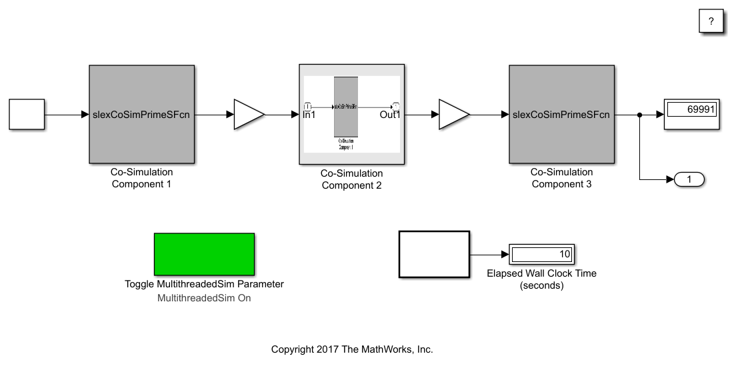This example shows how to run co-simulation on multiple threads.

Simulink is an integration platform that supports co-simulation between components with local solvers or that involves simulation tools. For example, co-simulation can involve an S-function as a co-simulation gateway between Simulink and third-party tools or custom code. It can also involve an FMU in co-simulation mode imported to Simulink.

This example shows how to run multithreaded co-simulation of three components (two implemented in C-MEX S-Function, one implemented using FMU Co-Simulation v2.0). These components compute prime numbers to find the maximum prime number that is smaller than or equal to the given input.

Multithreaded co-simulation best suits models with computationally intensive and loosely coupled components. In this example, each component computes prime numbers using a brute-force search. The intensity of the computation is directly proportional to the given range, which is a block input that you can experiment with. Also, components exchange a scalar signal, which is the maximum prime number within the given range, at communication times.

Experiment toggling the MultithreadedSim parameter and measuring the wall clock time.

```open_system('slexCoSimPrimeExample') sim('slexCoSimPrimeExample'); ```